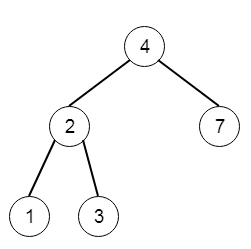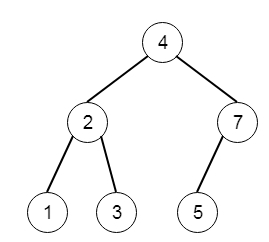# Insert into a Binary Search Tree in C++

Suppose we have a binary search tree. we have to write only one method, that performs the insertion operation with a node given as a parameter. We have to keep in mind that after the operation, the tree will remain BST also. So if the tree is like −if we insert 5, then the tree will be −To solve this, we will follow these steps −

• This method is recursive. this is called insert(), this takes a value v.
• if root is null, then create a node with given value v and make that as root
• if value of root > v, then
• left of root := insert(left of the root, v)
• else right of the root := insert(right of the root, v)
• return root

## Example(C++)

Let us see the following implementation to get a better understanding −

Live Demo

#include <bits/stdc++.h>
using namespace std;
class TreeNode{
public:
int val;
TreeNode *left, *right;
TreeNode(int data){
val = data;
left = right = NULL;
}
};
void insert(TreeNode **root, int val){
queue<TreeNode*> q;
q.push(*root);
while(q.size()){
TreeNode *temp = q.front();
q.pop();
if(!temp->left){
if(val != NULL)
temp->left = new TreeNode(val);
else
temp->left = new TreeNode(0);
return;
}
else{
q.push(temp->left);
}
if(!temp->right){
if(val != NULL)
temp->right = new TreeNode(val);
else
temp->right = new TreeNode(0);
return;
}
else{
q.push(temp->right);
}
}
}
TreeNode *make_tree(vector<int> v){
TreeNode *root = new TreeNode(v);
for(int i = 1; i<v.size(); i++){
insert(&root, v[i]);
}
return root;
}
void tree_level_trav(TreeNode*root){
if (root == NULL) return;
cout << "[";
queue<TreeNode *> q;
TreeNode *curr;
q.push(root);
q.push(NULL);
while (q.size() > 1) {
curr = q.front();
q.pop();
if (curr == NULL){
q.push(NULL);
}
else {
if(curr->left)
q.push(curr->left);
if(curr->right)
q.push(curr->right);
if(curr->val == 0 || curr == NULL){
cout << "null" << ", ";
}
else{
cout << curr->val << ", ";
}
}
}
cout << "]"<<endl;
}
class Solution {
public:
TreeNode* insertIntoBST(TreeNode* root, int val) {
if(!root)return new TreeNode(val);
if(root->val > val){
root->left = insertIntoBST(root->left, val);
}
else root->right = insertIntoBST(root->right, val);
return root;
}
};
main(){
Solution ob;
vector<int> v = {4,2,7,1,3};
TreeNode *root = make_tree(v);
tree_level_trav(ob.insertIntoBST(root, 5));
}

## Input

[4,2,7,1,3]
5

## Output

[4,2,7,1,3,5]